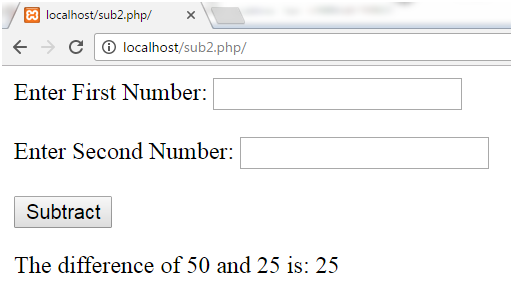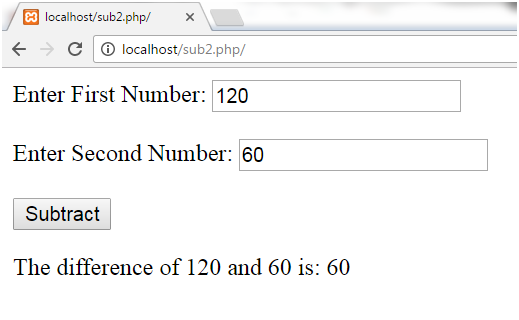Q:

# Subtracting Two Numbers in Php

There are three methods to subtract two numbers:

• Subtraction in simple code in PHP
• Subtraction in form in PHP
• Subtraction without using arithmetic operator (+).

## Subtraction in Simple Code

Subtraction of two numbers 30 and 15 is shown.

Example:

``````<?php
\$x=30;
\$y=15;
\$z=\$x-\$y;
echo "Difference: ",\$z;
?>  ``````

Output:## Subtraction in Form

By inserting values in the form two numbers can be subtracted.

Example:

``````<html>
<body>
<form method="post">
Enter First Number:
<input type="number" name="number1" /><br><br>
Enter Second Number:
<input type="number" name="number2" /><br><br>
<input  type="submit" name="submit" value="Subtract">
</form>
<?php
if(isset(\$_POST['submit']))
{
\$number1 = \$_POST['number1'];
\$number2 = \$_POST['number2'];
\$sum =  \$number1-\$number2;
echo "The difference of \$number1 and \$number2 is: ".\$sum;
}
?>
</body>
</html>  ``````

Output:## Subtraction in Form without (-) Operator

By inserting values in the form two numbers can be subtracted but without using (-) operator.

Example:

``````<body>
<form>
Enter First Number:
<input type="number" name="number1" /><br><br>
Enter Second Number:
<input type="number" name="number2" /><br><br>
<input  type="submit" name="submit" value="Subtract">
</form>
</body>
<?php

@\$number1=\$_GET['number1'];
@\$number2=\$_GET['number2'];
for (\$i=1; \$i<=\$number2; \$i++)
{
\$number1--;
}
echo "Difference=".\$number1;
?>  ``````

Output: